Hazard Ratios

The hazard ratio for a quantitative effect with regression coefficient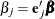is defined as exp, where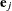denotes the jth unit vector. In general, a log-hazard ratio can be written as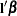, a linear combination of the regression coefficients, and the hazard ratio expis obtained by replacingwith.

The confidence intervals for hazard ratios are obtained by exponentiating the confidence limits of the corresponding linear combination. Thus, the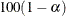confidence limits arewhere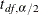is thepercentile point of the t distribution with df degrees of freedom. See the section Degrees of Freedom for more information about df. If you use the DF=NONE option in the MODEL statement, then the procedure uses thepercentile point of the standard normal distribution.